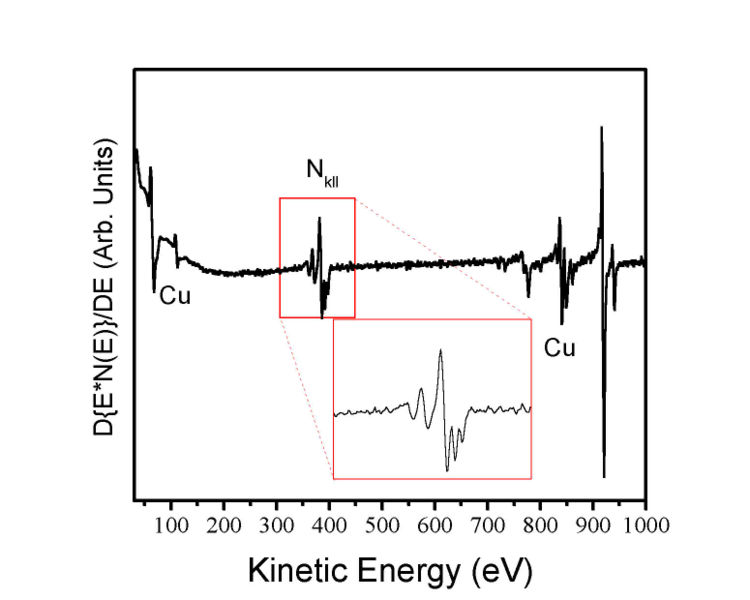# Auger Electron Spectroscopy

In Auger electron spectroscopy (AES) an incident electron beam results in the ionisation of a core atomic state. The excited atom can then relax through two competing effects: photon emission and electron emission. X-ray photon emission is favoured by the heavier elements, whilst Auger is dominant in the lower energy elements. The name of the process comes from its discoverer, Pierre Auger .

During photon emission the core hole is refilled by an electron and emits a photon with characteristic energy, this is the reverse of the photoelectric effect. The characteristic energy of this photon is the result of the electronic structure of the atom and can be used in compositional analysis. If this atom is then chemically bonded to another atom, the tendency of neighbouring atoms to attract electrons, their electronegativity, affects the energy levels within the atom under study. This shift in energy is called the chemical shift and allows for the determination o bonding environments.

In electron emission, intra-atomic relaxation occurs when an electron from an atomic orbital further from the nucleus fills the core hole. The energy liberated by this process results in the emission of another electron, the final atomic state is then doubly ionised. The final electron, named the Auger electron, has an energy determined by the atomic orbital it originated from. Figure 1 illustrates the process schematically.Figure 1: Diagram illustrating the Auger process. A photon ionises a core level, leaving a hole. The hole is filled by a higher energy electron and the subsequent energy is transferred to another electron, which then escapes.

Auger makes use of X-ray notation, where each atomic orbital (principal quantum number, n) is denoted by a letter (K, L, M, N equating to n = 1, 2, 3, 4) and the sub-shell is given a number. So that L1 is equivalent to the 2s shell and L2/L3 represent the spin-split 2p shell (2p1/2 and 2p3/2 respectively). As such, in Figure 1, the Auger process responsible for the final electron is denoted KL1L2,3 where K is the core hole, L1 is the relaxing electron's initial state and L2,3 is the initial state of the ejected electron. There are a variety of ejection processes, such as LMM and KVV. In a KVV process, a core level is ionised and valence electrons relax in order to fill the hole, as a result, these types of processes can be used to probe the local valence density of states. However, the many electron nature of this process makes this a very involved and complex procedure, limiting its usefulness relative to other valence state probes, for example UPS.

The energy of the emitted Auger electron is given by$KE = {E}_{K} - {{E}_{{L}_{1}}} - {{E}_{{L}_{2,3}}}$

and so gives information on the binding energies of the atomic species, allowing for identification of atomic species as well as chemical shifts.

Figure 2 illustrates a typical AES spectra, note that the left hand axis is plotted as the differential of the number of counts. Since the Auger process is relatively weak and the energies at which electrons are detected often coincides with the secondary electron background seen in photoelectron related spectroscopies, it is beneficial to take the derivative in order to highlight the Auger peaks relative to the flat background.Figure 2: Example AES spectra for copper nitride highlighting the nitrogen KLL peak. Image source: http://en.wikipedia.org/wiki/File:Cu3NAES.JPG

Consequently, Auger electrons can be used for compositional analysis in a similar fashion to that of XPS and is used in industry as an elemental characterisation technique. The primary advantage of AES is its surface sensitivity arising from the small mean free paths of the electrons emitted (between 200 and 3000 eV), however, energy losses within the solid tend to broaden the detected Auger signals. Often necessitating the use of XPS to resolve the chemical environments present before using AES to determine relative atomic quantities.

## References

1. D. P. Woodruff and T. Delchar (1994), Modern Techniques of Surface Science, Cambridge University Press

## Bibliography

• L. E. Davis, N. C. MacDonald, P. W. Palmberg, G. E. Rich and R. E. Weber (Eds.), Handbook of Auger Electron Spectroscopy, Physical Electronics Industries, Inc., 1976